# Wilson's Theorem

Theorem 

For any given graph$G$, there exists an integer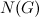$N(G)$ ($N(G)$ is exponentially large) such that if

•$n > N(G)$;
•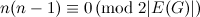$n(n-1) \equiv 0 \,({\rm mod \ }2|E(G)|)$; and
•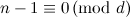$n-1 \equiv 0 \,({\rm mod \ }d)$ where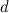$d$ is the greatest common divisor of the degrees of the vertices in$G$,

then there exists a$G$-design of order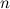$n$.

1. Wilson, R. M. Decompositions of complete graphs into subgraphs isomorphic to a given graph, Proceedings of the Fifth British Combinatorial Conference (Univ. Aberdeen, Aberdeen, 1975), Congressus Numerantium, No. XV, Utilitas Math., Winnipeg, Man. 647–659 (1976).